June 14, 2014

# Outline

• Motivation

• Graph representation options

• Inductive graphs

# Two aspects of graph representation

1. Representing graph structure

2. Representing typical graph algorithms

# Graph structure. Indirection

• Represent nodes as ints, edges as pairs

• Corresponds to usual definition of the graph as two sets

``````data Graph = Graph
{ nodes :: [Int]
, edges :: [(Int, Int)]
}

g1 = Graph
{ nodes = [1,2]
, edges = [(1,2), (2,1)]
}``````
• Easy to represent cycles.

• But:
• it is not an algebraic recursive data type as we'd expect
• no pattern matching like in lists or trees

# Graph structure, another approach

``````data Node a = Node a [Node a]
data Graph a = Graph [Node a]``````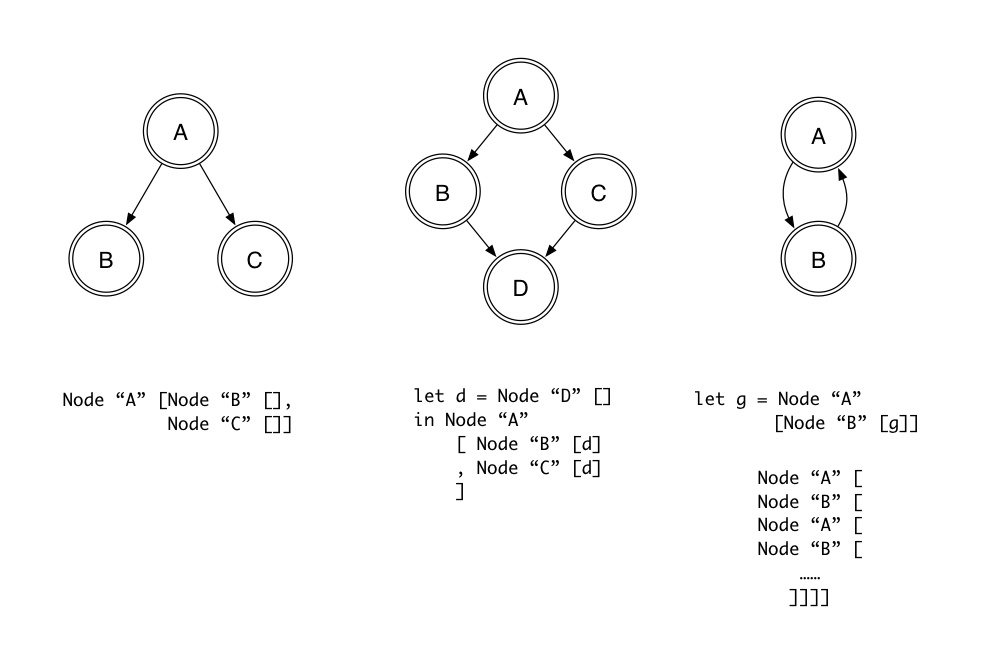# Graph structure

• Not so fast. Cycles are tricky. Sharing is tricky.

• We can represent DAGs using sharing, but this sharing is not observable.

• We can represent cycles using Haskell laziness and "tying the knot" technique, but it is fragile.

# Graph algorithms

• First intuitive idea: mimicking imperative graph algorithms

• Imperative algorithms usually maintain additional data structure (e.g. to mark visited nodes)

• But:
• we don't have convenient arrays with O(1) write
• maintaining state is somewhat painful
• Can we do better?

# Inductive graphs idea

• Introduced in "Inductive graphs. Functional graph algorithms" paper by Martin Erwig.

• Limitations of previous approaches are caused by monolotic representation of graphs. The algorithms are bulky and need to keep track additional information regarding to traversal.

• Algorithms on lists and trees are elegant and easy to reason about.

• Inductive graphs: an inductive data structure with two constructors similar to lists or trees.

• Profit: we can use pattern matching and immediately express and reason about simple algorithms (reversing the edges, calculating nodes, etc.).

• This idea has been implemented in "fgl" library by Martin Erwig included in Haskell platform. FGL = functional graph library.

# Types

``````type Node = Int
type Adj e = [(e, Node)]

data Graph v e
= Empty
| Context v e :&: Graph v e``````
• Akin to lists we either have an empty graph or an extension of the graph with a context

• Context v e: v and e are types for labels (for vertices and edges)

• Context represents a node, its label and its inbound and outbound edges

# Context example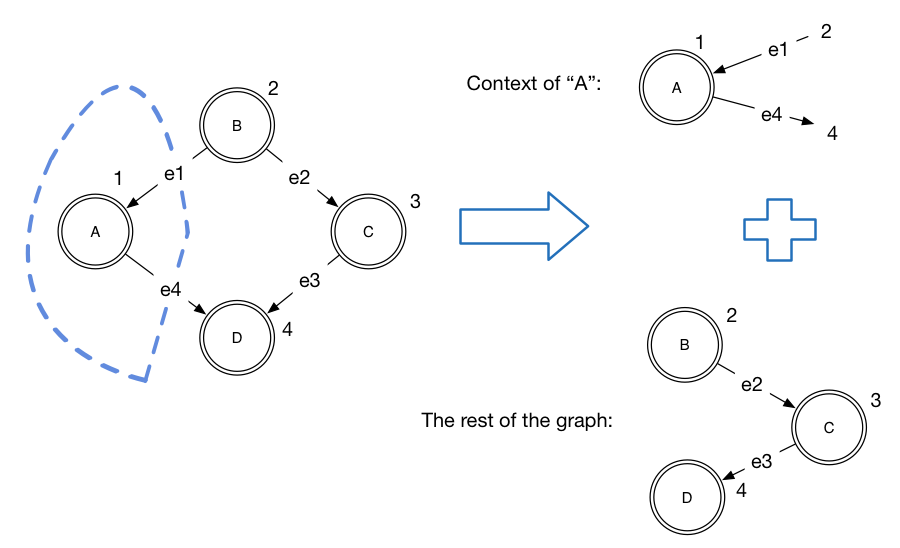``context of node 1 = ([(2, "e1")], 1, "A", [(4, "e4")])``

# Contexts order

• The order of inserting contexts is not unique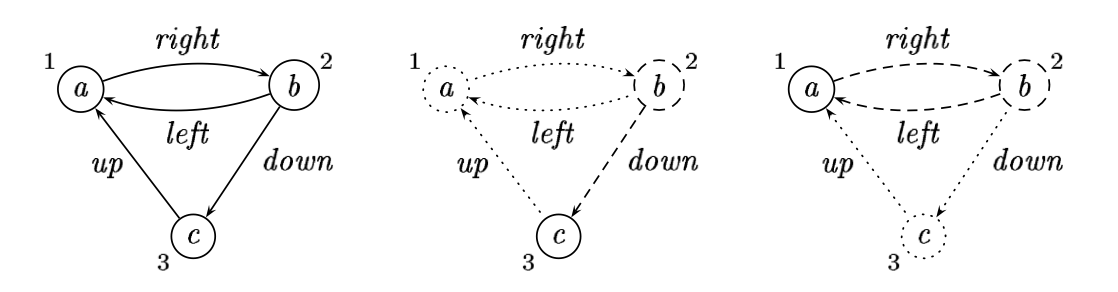``````([("left", 2), ("up", 3)], 1, 'a', [("right", 2)])
&
([], 2, 'b', [("down", 3)])
&
([], 3, 'c', [])
&
Empty``````

# Pattern matching on graphs

• Inductive structures give pattern matching
``````isEmpty :: Graph v e -> Bool
isEmpty Empty = True
isEmpty _ = False

gmap :: (Context v1 e1 -> Context v2 e2) -> Graph v1 e1 -> Graph v2 e2
gmap _f Empty = Empty
gmap f (c :&: g) = f c :&: gmap f g

grev :: Graph v e -> Graph v e
grev = gmap swap where swap (i, n, v, o) = (o, n, v, i)``````

# Proofs

• Using this style it is easy to prove things
``````grev (grev g) = g

gmap f . gmap g = gmap (f . g)             (1)
swap . swap = id                           (2)``````

# Unordered fold and its usage

``````ufold :: (Context v e -> acc -> acc) -> acc -> Graph v e -> acc
ufold _ acc Empty = acc
ufold f acc (c :&: g) = f c (ufold f acc g)

gmap = ufold (\c -> (f c :&:)) Empty

nodes :: Graph v e -> [Node]
nodes = ufold (\(_,v,_,_) -> (v:)) []

undir :: Graph v e -> Graph v e
undir = gmap (\(p,v,l,s) -> let ps = nub (p ++ s) in (ps,v,l,ps))``````

# Pattern matches

• For efficiency reasons Graph is an abstract type, so we have to use a smart constructor (&) instead of constructor (:&:)

• To facilitate this we have the following functions:

``````isEmpty :: Graph v e -> Bool

-- matchAny extracts any context from the graph
matchAny :: Graph v e -> (Context v e, Graph v e)

-- unordered graph handling idiom
gmap f g | isEmpty g = g
| otherwise = f c & (gmap f g')
where (c, g') = matchAny g``````

# Ordered pattern matches

``````-- match decomposes a graph into a context for particular node and the rest of the graph
match :: Node -> Graph v e -> (Maybe (Context v e), Graph v e)

-- usage example
f v g = case match v g of
(Nothing, g') -> e1
(Just c, g')  -> e2
``````

# Example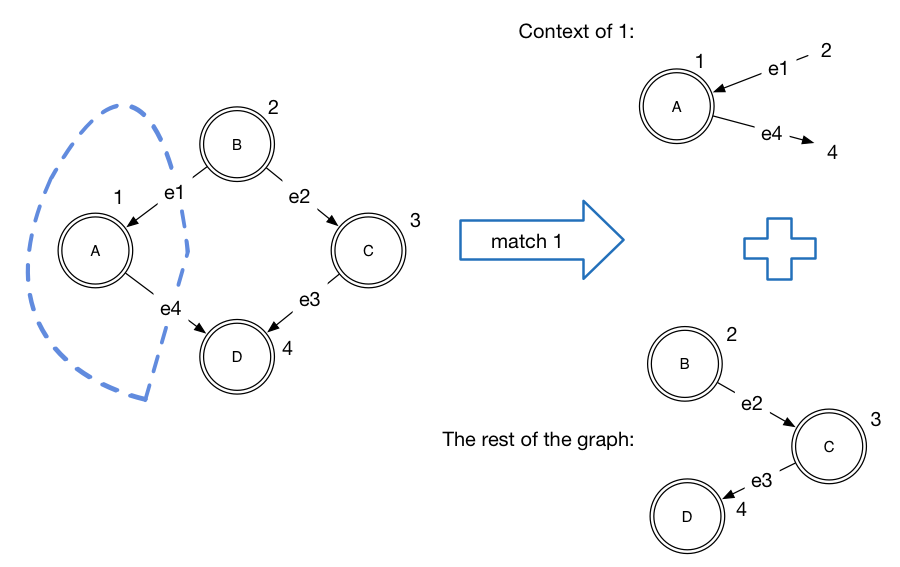# Functional graph algorithms. DFS.

• Depth first search: useful algorithm which is a base for many other algorithms (topological sort, SCC, etc.)

• Idea: visit each node in a graph once by visiting successors before siblings.

• Input: we provide a list of nodes, it is needed if graph contains several components.

• Output: a list of nodes in traversal order.

``````dfs :: [Node] -> Graph v e -> [Node]
dfs [] _ = []
dfs (v:vs) g = case match v g of
(Just c, g') = v : dfs (suc c ++ vs) g'
(Nothing, g') -> dfs vs g'``````
• Note how we use decomposition of graph with `match` to avoid visiting the same node more than once. When context of the node is matched, we return the rest of the graph which does not contain this node anymore.

# DFS to BFS.

• It's quite easy to turn DFS into BFS.
``````dfs :: [Node] -> Graph v e -> [Node]
dfs [] _ = []
dfs (v:vs) g = case match v g of
(Just c, g') = v : dfs (suc c ++ vs) g'
(Nothing, g') -> dfs vs g'``````

# DFS to BFS.

• It's quite easy to turn DFS into BFS.
``````bfs :: [Node] -> Graph v e -> [Node]
bfs [] _ = []
bfs (v:vs) g = case match v g of
(Just c, g') = v : bfs (vs ++ suc c) g'
(Nothing, g') -> bfs vs g'``````

# DFS to BFS.

• It's quite easy to turn DFS into BFS.
``````bfs :: [Node] -> Graph v e -> [Node]
bfs [] _ = []
bfs (v:vs) g = case match v g of
(Just c, g') -> v : bfs (vs ++ suc c) g'
(Nothing, g') -> bfs vs g'``````
• However, using lists for queues is inefficient, so practically BFS is implemented using better queues (with O(1) enqueue and dequeue)

# DFF

• DFF = depth first search spanning forest.

• DFF is a forest (list of tree) which contain nodes in the order of depth first search traversal.

• DFF is used in topological sort and strongly connected components algorithms

``````data Tree a = Br a [Tree a]

dff :: [Node] -> Graph v e -> [Tree Node]``````

# DFF example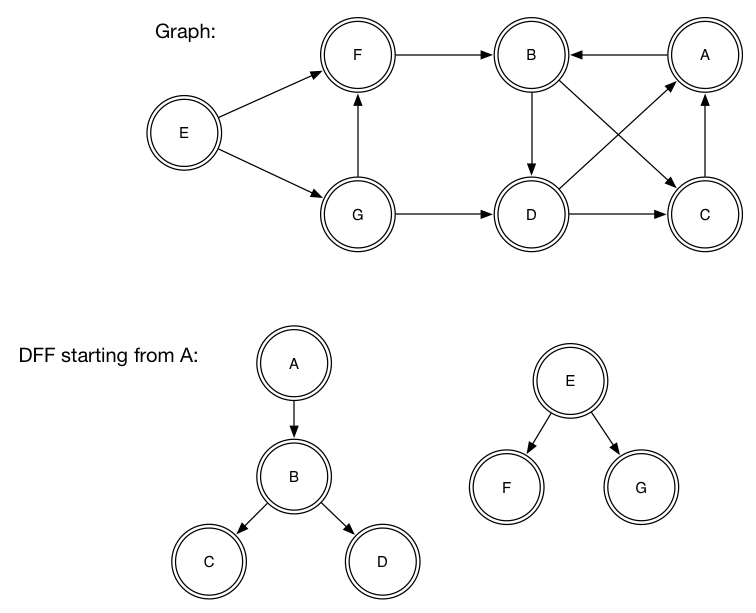# DFS-based algorithms

• We compose functions, instead of changing/tuning the DFS routine (as it is usually done in imperative algorithms)
``````-- Toppological sort is a reversed post-order on DFS
topsort :: Graph v e -> [Node]
topsort = reverse . concatMap postOrder . dff

-- Kosaraju-Sharir algorithm for strongly connected components
scc :: Graph v e -> [Tree Node]
scc g = dff (topsort g) (grev g)``````
• Nice and elegant!

# Complexity and internals

• There are several implementations: based on trees and Patricia trees (Data.IntMap).

• We'd like to have composition and decomposition of the graph to be constant or at least sublinear.

• Data.IntMap insert, delete and adjust has O(min(log n, W)) worstcase where W is number of bits in Int (32 or 64).

• Composition and decomposition of the graph (& and match) are still linear in terms of the vertex degree, since we have to handle all edges.

• Overall, theoretical complexity of provided implementations are not great, but according to the paper works well in practice.

# Other algorithms in FGL

• 18 (!) flavours of DFS/DFF (parameterized by pretty much everything)
• Topological sort/SCC/reachable and other algorithms based on DFS
• BFS
• Biconnected components
• Minimum spanning trees
• Shortest paths
• Maximum flows
• Articulation points
• Dominators
• Voronoi diagrams
• Transitive closures

# FGL on hackage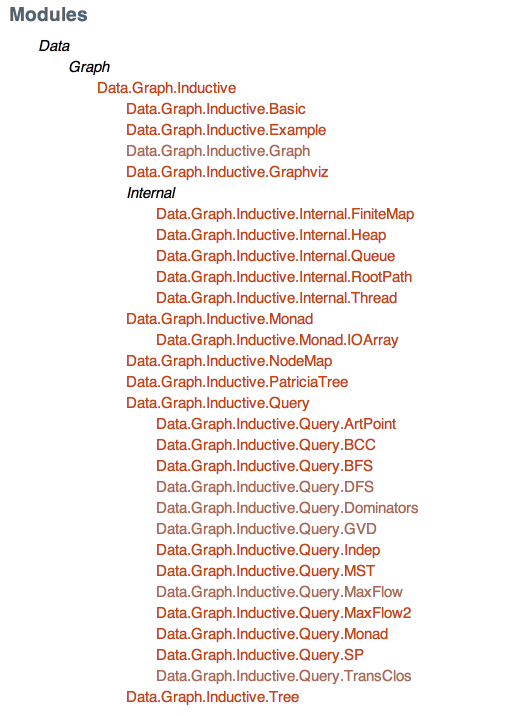# Summary

• The major selling point of FGL is its API

• FGL provides rich and convenient API a la algebraic data types

• API facilitates expressing graph algorithms in terms of recursive definitions

# Other graph libraries

• Data.Graph from "containers" package
• some minimalist library from Edward Kmett
• libraries for specialized graphs